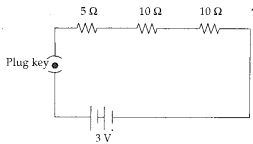# Draw a schematic diagram of an electric circuit consisting of a battery of two cells each of 1.5V, 5Ω, 10Ω and 15Ω

Draw a schematic diagram of an electric circuit consisting of a battery of two cells each of 1.5V, 5Ω, 10Ω and 15Ω resistors and a plug key, all connected in series.

The emf of each cell is 1.5V.
When the two cells are connected in series, the emf of the battery becomes (1.5 + 1.5) V = 3V.
Therefore, the schematic diagram of the series circuit is plug key as given figure.